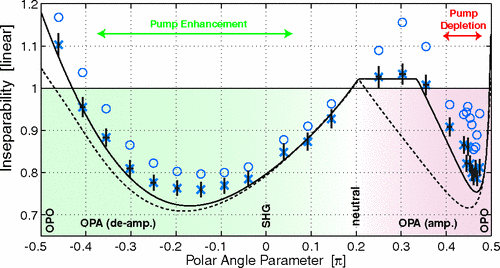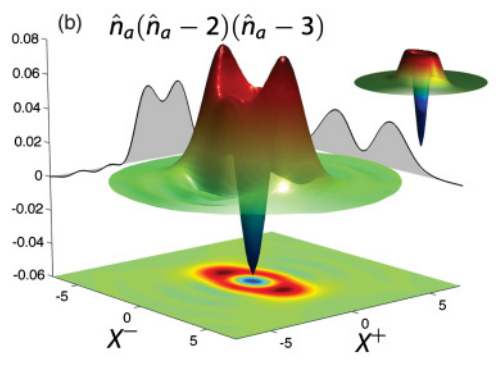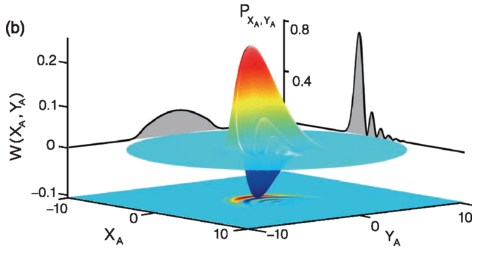## Non classical states

A core part of our research is to produce and characterise non-classical states of light. There are numerous interpretations on what non-classicality means. Here is a list of some of the things that we have done.

#### Squeezing

The ANU quantum optics group has a long history in generating squeezed light. In 2004, Kirk McKenzie demonstrate the generation of broadband continuous-wave optical squeezing from 280 Hz to 100 kHz . This low frequency squeezing is ideal for improving the sensitivity of audio frequency measuring devices such as gravitational wave detectors. In 2018, Francesco Lenzini and Jiri Janousek measured 1.4 dB of squeezing in an integrated photonic platform . We also demonstrate entanglement by verifying an inseparability criterion of 0.77. In 2022, Biveen Shajilal demonstated 12.6 dB squeezing at 1550 nm. This setup delivered greater than 10 dB of squeezing for over 50 hours .

#### Photon anti-bunching

The traditional way for measuring the second-order coherence function of light is by using a Hanbury-Brown Twiss intensity interferometer. In 2007, Nicolai Grosse presented a technique for measuring the second-order coherence function using homodyne detection . The second-order coherence of a displaced squeezed state was measured and the best anti-bunching statistics of 0.11 was observed.

#### Harmonic entanglementIn 2006, Nicolai Grosse investigate using the second-order non-linear interaction as a means to generate entanglement between fields of differing wavelengths. He show that perfect entanglement can be produced between the fundamental and second harmonic fields in these processes . In 2008, Nicolai Grosse experimentally demonstrated entanglement between two beam separated by an octave in frequency .

#### Non-Gaussian statesIn 2011, Helen Chrzanowski used a homodyne conditional measurement to simulate the statistics of a photon number discrimination measurement to reconstruct a non-Gaussian state . This setup was improved in 2013 by phase randomising the homodyne measurements . This is possible because the Fock states are phase invariant.

#### Discord

In 2012, Mile Gu and Helen Chrzanowski demonstrated the operational significance of discord . The amount of discord consumed in encoding information can only be accessed fully by coherent quantum interactions. The inability to access this information by any other means allow us to use discord to directly quantify this quantum advantage. In 2014, Sara Hosseini introduced and experimentally demonstrate an efficient technique to verify quantum discord in unknown Gaussian states .

In 2018, Mark Bradshaw examined the quantum discord of a multipatite state in the experimentally relevant case when the quantum states are Gaussian and the measurements are restricted to Gaussian measurements . We find that by adding uncorrelated noise and multiplicative noise, the quantum discord always decreases. However, correlated noise can either increase or decrease the quantum discord. We also find that for low noise, the optimal classical measurements are single quadrature measurements. As the noise increases, a dual quadrature measurement becomes optimal.

#### Nonlinear entanglementTraditional entanglement describes linear correlations between two objects in terms of pairs of conjugate observables. In 2015, Shen Yong showed that with a third-order nonlinear interaction, the correlations between the two objects can now be highly nonlinear . This can be used to generate the [cat state]](nonlinear_entanglement.mp4) or even the Sydney opera house state.

#### Continuous variable Bell violationA Bell inequality is a fundamental test to rule out local hidden variable model descriptions of correlations between two physically separated systems. There have been a number of experiments in which a Bell inequality has been violated using discrete-variable systems. In 2018, Oliver Thearle demonstrate a violation of Bells inequality using continuous variable quadrature measurements . By creating a four-mode entangled state with homodyne detection, we recorded a clear violation with a Bell value of 2.31. This opens new possibilities for using continuous variable states for device independent quantum protocols.

#### References

 K. McKenzie et al., “Squeezing in the audio gravitational-wave detection band,” Physical review letters, vol. 93, no. 16, p. 161105, 2004.

 F. Lenzini et al., “Integrated photonic platform for quantum information with continuous variables,” Science advances, vol. 4, no. 12, p. eaat9331, 2018.

 N. B. Grosse, T. Symul, M. Stobińska, T. C. Ralph, and P. K. Lam, “Measuring photon antibunching from continuous variable sideband squeezing,” Physical review letters, vol. 98, no. 15, p. 153603, 2007.

 N. B. Grosse, W. P. Bowen, K. McKenzie, and P. K. Lam, “Harmonic entanglement with second-order nonlinearity,” Physical review letters, vol. 96, no. 6, p. 063601, 2006.

 N. B. Grosse, S. Assad, M. Mehmet, R. Schnabel, T. Symul, and P. K. Lam, “Observation of entanglement between two light beams spanning an octave in optical frequency,” Physical review letters, vol. 100, no. 24, p. 243601, 2008.

 H. M. Chrzanowski et al., “Photon-number discrimination without a photon counter and its application to reconstructing non-Gaussian states,” Phys. Rev. A, vol. 84, no. 5, Nov. 2011.

 H. M. Chrzanowski et al., “Reconstruction of photon number conditioned states using phase randomized homodyne measurements,” J. Phys. B: At., Mol. Opt. Phys., vol. 46, no. 10, p. 104009, May 2013.

 M. Gu et al., “Observing the operational significance of discord consumption,” Nature Physics, vol. 8, no. 9, p. 671, 2012.

 S. Hosseini et al., “Experimental verification of quantum discord in continuous-variable states,” Journal of Physics B: Atomic, Molecular and Optical Physics, vol. 47, no. 2, p. 025503, 2014.

 M. Bradshaw, P. K. Lam, and S. M. Assad, “Gaussian multipartite quantum discord from classical mutual information,”Journal of Physics B: Atomic, Molecular and Optical Physics, Vol. 52, No. 24, p. 245501, 2019.

 Y. Shen, S. M. Assad, N. B. Grosse, X. Y. Li, M. D. Reid, and P. K. Lam, “Nonlinear entanglement and its application to generating cat states,” Physical review letters, vol. 114, no. 10, p. 100403, 2015.

 O. Thearle et al., “Violation of bell’s inequality using continuous variable measurements,” Physical review letters, vol. 120, no. 4, p. 040406, 2018.

 B. Shajilal, O. Thearle, A. Tranter, Y. Lu, E. Huntington, S. Assad, P. K. Lam, and J. Janousek. "12.6 dB squeezed light at 1550 nm from a bow-tie cavity for long-term high duty cycle operation," Optics Express 30, no. 21: 37213-37223, 2022.